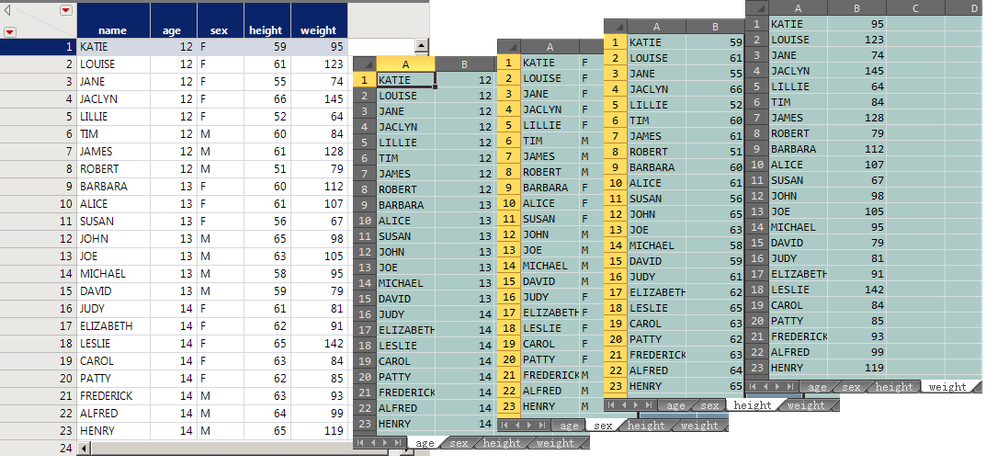Our World Statistics Day conversations have been a great reminder of how much statistics can inform our lives. Do you have an example of how statistics has made a difference in your life? Share your story with the Community!
Choose Language Hide Translation Bar
Highlighted

## How to used JSL JMP tables into a multi-tabbed Excel workbook？

I would like to ask JMP community to help me out on a custom way to export JMP table to excel.

Thanks!``dt = Open( "\$SAMPLE_DATA/Big Class.jmp" );``
1 ACCEPTED SOLUTION

Accepted Solutions
Highlighted

## Re: How to used JSL JMP tables into a multi-tabbed Excel workbook？

I am not sure exactly what all you need with your code, but here is a simple script that creates your required subsets and saves them to an Excel workbook.  If you need to save the jmp tables as separate tables, in addition to the workbook, you can do that with a simple loop after the workbook is created.

``````Names Default To Here( 1 );
dt = Open( "\$SAMPLE_DATA/Big Class.jmp" );

cNa = dt << get column names;
xF = {};
For( c = 2, c <= N Col( dt ), c++,
d2 = dt << Subset(
All rows,
columns( dt:name, Column( dt, c ) ),
output table( Char( cNa[c] ) )
);
Insert Into( xF, d2 << get name );
);

close( dt, nosave );

Create Excel Workbook( "c:\TheWorkBook.xlsx", Eval( xF ), Eval( xF ) );``````
Jim
11 REPLIES 11
Highlighted

## Re: How to used JSL JMP tables into a multi-tabbed Excel workbook？

Hi @lwx228,

One resource within JMP that is very helpful is the Scripting Index platform under Help.

You'll want to use the Save As on your data table. After you've opened it and done whatever you want to it, you would do something like:

``dt << Save As("Filepath\filename.xlsx")``

You can have all sorts of extensions, csv, txt, etc. It then saves it according to the extension.

You can even create an Excel workbook with somethign like:

``Create Excel Workbook("filepath\filename.xlsx",{"data table name 1", "data table name 2"}, {"Worksheet name 1", "Worksheet name 2"})``

It sounds like what you want to do is something more like the last option.

Hope this helps!,

DS

Highlighted

## Re: How to used JSL JMP tables into a multi-tabbed Excel workbook？

I still don't get it.Not done.
Thanks!

``Create Excel Workbook( "c:\MyWorkbook.xlsx", {"Big Class", "Abrasion"}, {"Big", "Abrasive"} );``
Highlighted

## Re: How to used JSL JMP tables into a multi-tabbed Excel workbook？

This example should work in JMP 13 or later

``````names default to here(1);

open("\$SAMPLE_DATA/big class.jmp");
open("\$SAMPLE_DATA/airline delays.jmp");

Create Excel Workbook(
"\$TEMP\TheWorkBook.xlsx",
{"big class", "Airline delays"},
{"Big", "Airline"}
);

wait(0);
close(data table("Big Class"), nosave);
close(data table("airline delays"), nosave);

wait(4);
open("\$TEMP\TheWorkBook.xlsx");``````
Jim
Highlighted

## Re: How to used JSL JMP tables into a multi-tabbed Excel workbook？

Thank Jim!

My requirements differ from the code of the JMP sample.

I opened only one JMP file and divided "age, sex, height and weight" in the “Big class" into 4 different tables with name respectively, and saved them into 4 different tables in the same excel workbook.The four tables in this excel workbook are named "age," "sex," "height," and "weight."

Thanks!
Highlighted

## Re: How to used JSL JMP tables into a multi-tabbed Excel workbook？

I provided the above code as a complete example that works on my system for both JMP 13 and JMP 14. So if you can run the code that I provided, it would give you an indication on where your problem might be. And as far as JMP and Excel are concerned, a data table is just a data table, so where they come from should not matter.
Jim
Highlighted

## Re: How to used JSL JMP tables into a multi-tabbed Excel workbook？

I understand. First, divide "Big class.jmp "file into 4 JMP files with JSL as required, and then run the above code to generate an excel file of 4 tables.

Thank Jim!
Highlighted

## Re: How to used JSL JMP tables into a multi-tabbed Excel workbook？

``````dt = Current Data Table();di="c:\";
cNa=dt<<get column names;xF=As List(cNa[2 ::ncol(dt)]);
for(c=2,c<=ncol(dt),c++,
current data table(dt);ca=Column(c)<<Get Name;
d2 =dt<< Subset( All rows, columns( :name, Column(c) ) );Wait(0);
d2<<Save(di ||ca ||".jmp" );
);
dt<<Close Window;
Create Excel Workbook("c:\TheWorkBook.xlsx",xF,xF);``````

xF is

{"","","",""}

{,,,}

Highlighted

## Re: How to used JSL JMP tables into a multi-tabbed Excel workbook？

You need to evaluate the list:

``````names default to here(1);

open("\$SAMPLE_DATA/big class.jmp");
open("\$SAMPLE_DATA/airline delays.jmp");

dts = {"big class", "Airline delays"};

Create Excel Workbook(
"\$TEMP\TheWorkBook2.xlsx",
eval( dts )
,
);

wait(0);
close(data table("Big Class"), nosave);
close(data table("airline delays"), nosave);

wait(4);
open("\$TEMP\TheWorkBook2.xlsx");``````
Jim
Highlighted

## Re: How to used JSL JMP tables into a multi-tabbed Excel workbook？

I understand the full JSL is like this.

But can't it ——{"age","sex","height","weight"}——be generated automatically by code?This enables automation for different files.

Thanks!

``````dt = Open( "\$SAMPLE_DATA/Big Class.jmp" );
dt = Current Data Table();di="c:\";
cNa=dt<<get column names;xF=As List(cNa[2 ::ncol(dt)]);
for(c=2,c<=ncol(dt),c++,
current data table(dt);ca=Column(c)<<Get Name;
d2 =dt<< Subset( All rows, columns( :name, Column(c) ) );Wait(0);
d2<<Save(di ||ca ||".jmp" );
);
dt<<Close Window;
Create Excel Workbook("c:\TheWorkBook.xlsx",{"age","sex","height","weight"},{"age","sex","height","weight"});``````

Article Labels

There are no labels assigned to this post.